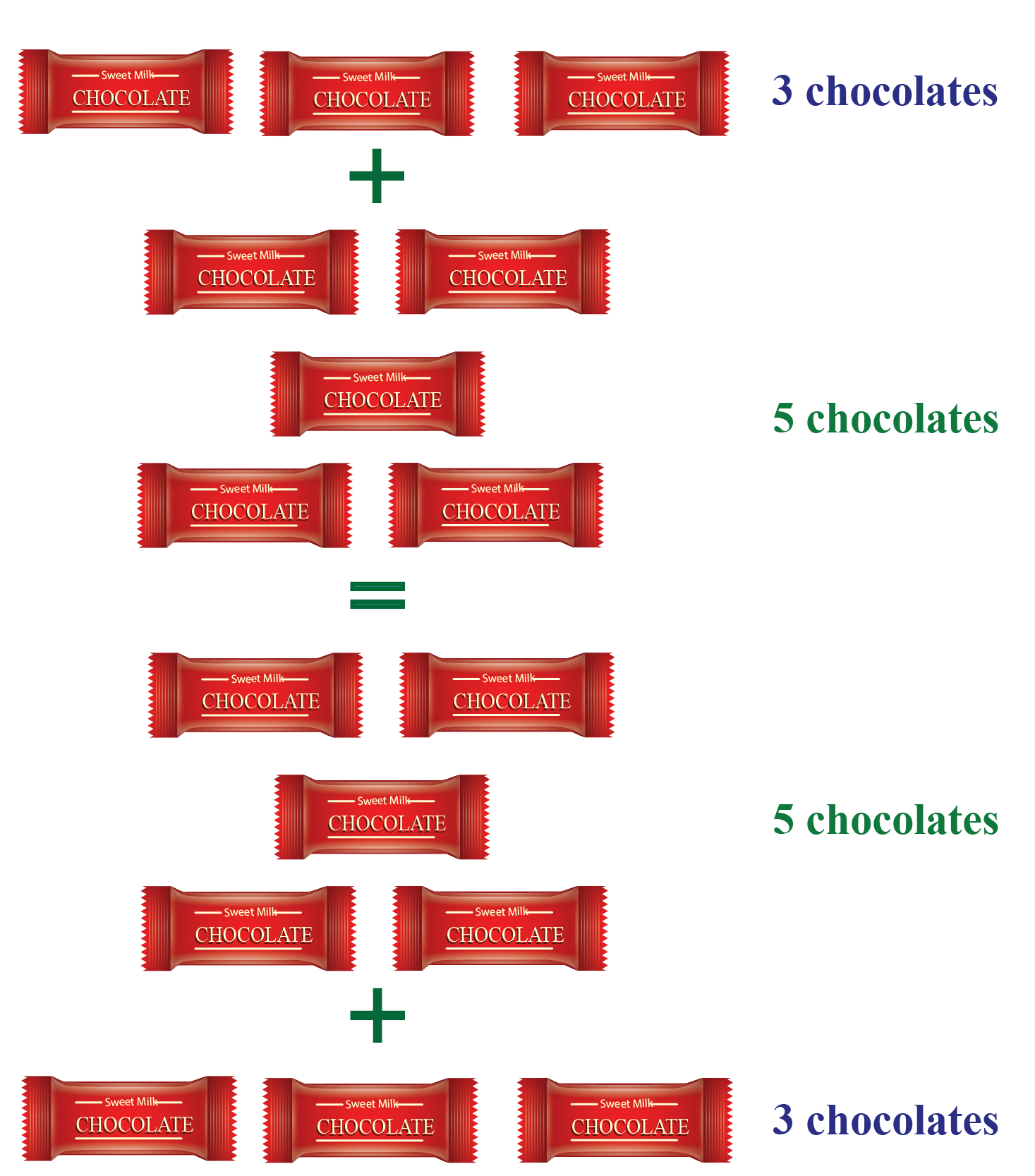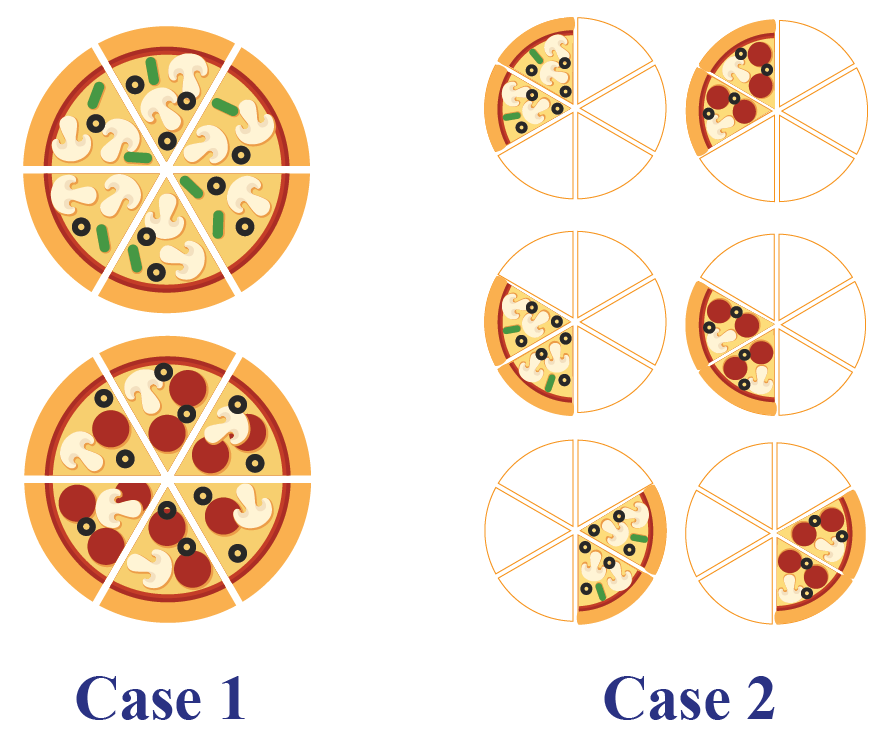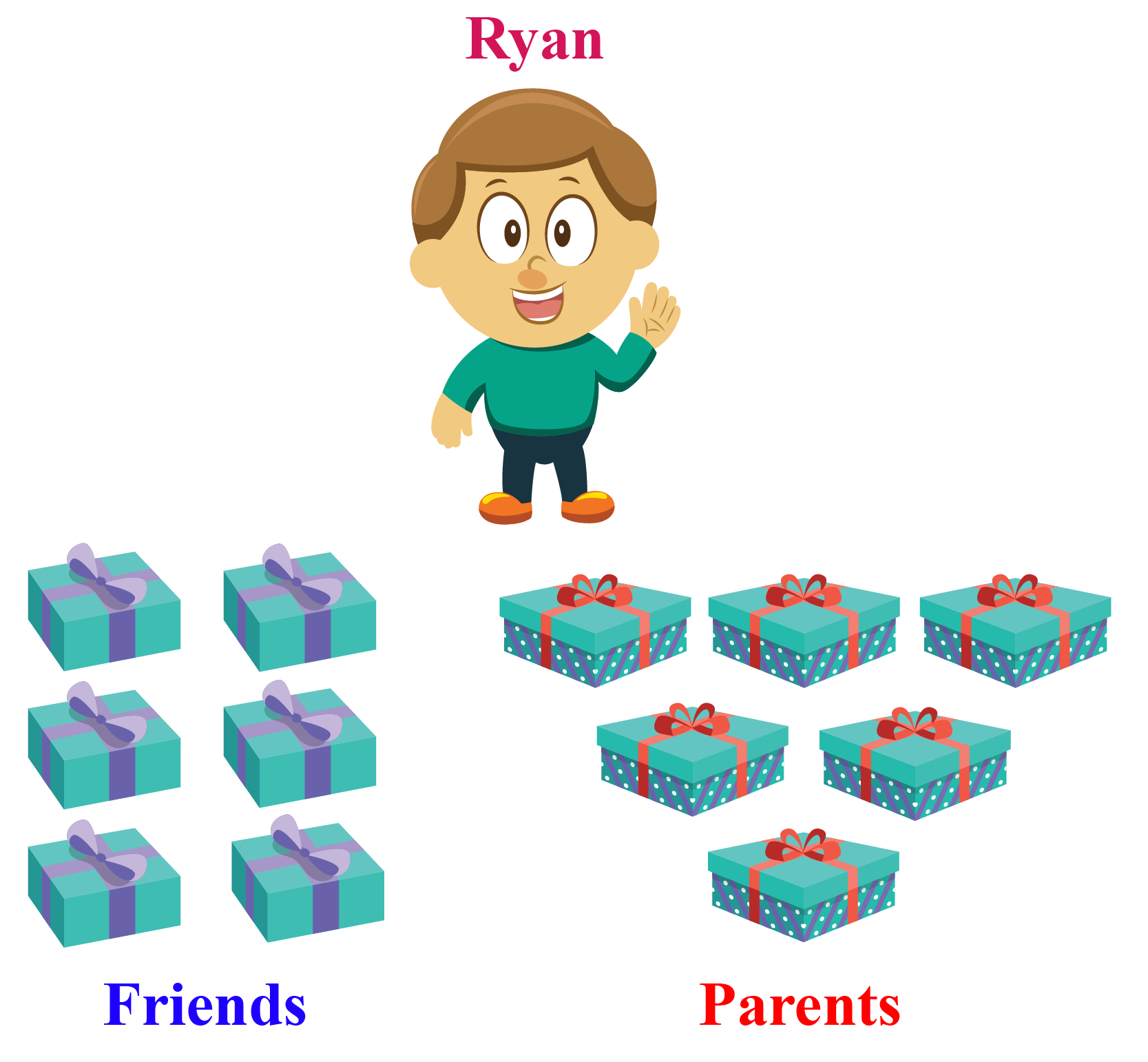# Commutative Property

In this mini-lesson, we will explore the world of the commutative property. We will do so by finding what is the definition of commutative property, how to apply it in addition and multiplication numbers, and so much more.

And not to forget, we will also discover the interesting facts around them.

You will get a chance to play with interactive simulations to know more about the lesson and can try your hand at solving a few interesting practice questions at the end of the page.

## Lesson Plan

 1 What Is Commutative Property? 2 Important Notes on Commutative Property 3 Solved Examples on Commutative Property 4 Challenging Questions on Commutative Property 5 Interactive Questions on Commutative Property

## What Is Commutative Property?

The word "Commutative" originates from the word "commute," which means "to move around".

Hence, the Commutative Property deals with moving the numbers around.

So mathematically, we can define any binary operation as a commutative one, if changing the order of the operands does not change the result of the operation.

### Definition

The Commutative Property of addition says that changing the order of the addends will not change the value of summation.Given below is a solved example for the Commutative Property of Addition.

Example:

Sam was given 3 chocolates by his mother for his good behavior, and 5 chocolates by his father for cleanliness.

He wants to know the total number of chocolates he has got.

Sam knows that 5+3=8

Does commutative property apply while finding the total number of chocolates Sam has?Solution:

By simple rules of addition, we can say that the total number of chocolates with Sam can be calculated as:

Total Chocolates = Chocolates by Mother + Chocolates by Father

$$\therefore$$ Total number of chocolates = 3+5

Now, Sam knows that 5+3=8, so we try to rearrange the number of chocolates he has according to this equation.

We can say that by using commutative property, the total number of chocolates can be found as 3+5 = 5+3. Hence, we can say that

Total Chocolates = Chocolates by Father + Chocolates by Mother

$$\therefore$$ Total number of chocolates = 5+3

But we already know that 5+3=8

 Thus, the total number of chocolates is 8. $$\therefore$$ Commutative property applies.

## Commutative Property of Multiplication

### Definition

The Commutative Property of Multiplication says that the order in which we multiply the numbers does not change the final product.Given below is a solved example for the Commutative Property of Multiplication.

Example:

Richard cuts a pizza into 6 slices, and he eats a total of 2 pizzas.

How many slices of pizza does he eat?

Will it be the same if he has 2 slices from each pizza from a total of 6 pizzas?Solution

Let us find the total number of slices of pizza eaten by Richard in both cases.

Case 1) The total number of slices can be found as

2 Pizzas $$\times$$ 6 Slices each = 12 Slices total

Case 2) The total number of slices can be found as

6 Pizzas $$\times$$ 2 Slices each = 12 Slices total

 $$\therefore$$ Total number of slices in both cases is $$12$$. Hence, it is the same for both cases.

Now, try applying what you learned till now in the simulation given here. Write an equation and get its Commutative right beside it! Try using addition as well as multiplication, and cross verify your answers!

## Solved Examples

 Example 1

Before going to school, Jacky's mother asked him whether $$x+y=y+x$$ is an example of the commutative property.

Can you help Jacky find out whether it is commutative or not?

Solution

We know that commutative property states that changing the order of the addends will not change the value of summation.

So, we see that changing the order will not alter the summation value.

Hence, the summation remains same. So this is an example of commutative property.

 $$\therefore$$ This is an example of commutative property.
 Example 2

Benny was given a question in his assignment, which asked to solve the equation 3+5+7=15 commutatively.

Can you help Bran with his assignment?

Solution

By simple rules of addition, we can say that the final summation can be calculated as:

Summation $$= 3+7+5=15$$

$$\therefore$$ The summation value is unchanged.

Now, we can also say that:

Summation $$= 5+3+7=15$$

$$\therefore$$ The summation value is still unchanged.

In both the cases, our answer for the final summation is the same.

 $$\therefore$$ Commutative property applies.
 Example 3

Today is Ryan's birthday party.

Three of his friends are bringing 2 gifts each for him, while both of his parents are bringing 3 gifts each for him.

Is the number of gifts the same in both cases?

Which property will you use to show this?Solution

Let us calculate the total number of gifts in both cases.

Case 1) The total number of gifts can be calculated as

3 Friends $$\times$$ 2 Gifts each = 6 Gifts

Case 2) The total number of gifts can be calculated as

2 Parents $$\times$$ 3 Gifts each = 6 Gifts

$$\therefore$$ Total number of gifts in both cases is $$6$$

We know that $$2 \times 3 =3 \times 2 = 6$$ is nothing but changing the order of the numbers which are multiplied.

Hence, this is an example of the Commutative Property of Multiplication.

 $$\therefore$$ Commutative Property of Multiplication is proven.
 Example 4

Comment whether the given situations are commutative or not:

Solution

By simple rules of addition, we can say that the total number of apples in your bag can be calculated as:

Total apples = Initial apples + Added apples  $$= 3+6=9$$ apples

$$\therefore$$ Total number of apples is 3+6 = 9 apples.

Now, for second case we can say that:

Total apples = Initial apples + Added apples  $$= 6+3=9$$ apples

$$\therefore$$ Total number of apples is 6+3 = 9 apples.

Thus, in both cases, our answer for the total number of apples is the same.

 $$\therefore$$ Commutative Property applies.
 Example 5

Rinny walked 5 miles to the North, and then 3 miles to the East.

Will she end up at the same place if she would have walked 3 miles to the East first, and then 5 miles to the North?

Solution

We try to convert this on a coordinate plane.

We assume the miles he walked to the North to be the y-coordinate, while miles in the East to be the x-coordinate.

By simple rules of vector addition, we can say that the final position of Rinny can be calculated as:

Final position 1 = 5 miles to the North + 3 miles to the East $$=(3,5)$$

$$\therefore$$ The final coordinate =(3,5)

Now, we can also say that:

Final position 2 = 3 miles to the East + 5 miles to the North $$=(3,5)$$

$$\therefore$$ The final coordinate =(3,5)

Thus, in both the cases, our answer for the final position coordinate is the same.

 $$\therefore$$ Rinny will end up at the same place.Challenging Questions
• Mark's teacher gave him 3 stars for punctuality, and 4 stars for creativity in his homework notebook. The next day, his teacher again gave him 5 stars for punctuality, and 2 stars for creativity. He noticed that he received a total of 7 stars each day in total from his teacher.
Is this an example of commutative property of addition? Why do you think so?

## Interactive Questions

Here are a few activities for you to practice.

## Let's Summarize

We hope you enjoyed learning about the commutative property with the simulations and practice questions. Now, you will be able to easily solve problems on the commutative property of addition and the commutative property of multiplication.

At Cuemath, our team of math experts is dedicated to making learning fun for our favorite readers, the students!

Through an interactive and engaging learning-teaching-learning approach, the teachers explore all angles of a topic.

Be it worksheets, online classes, doubt sessions, or any other form of relation, it’s the logical thinking and smart learning approach that we, at Cuemath, believe in.

## 1. What is the difference between commutative property and associative property?

Commutative property states that A + B = B + A and on the other hand, associative property states that (A + B) + C = A + (B + C).

## 2. What are commutative laws?

The commutative laws are  $$A+B=B + A$$ and $$A \times B=B \times A$$.

More Important Topics
Numbers
Algebra
Geometry
Measurement
Money
Data
Trigonometry
Calculus